Next: Bayes' theorem Up: Conditional probability and Bayes' Previous: Dependence of the probability   Contents

## Conditional probability

Although everybody knows the formula of conditional probability, it is useful to derive it here3.5. The notation is, to be read probability of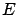given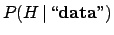'', wherestands for hypothesis. This means: the probability thatwill occur under the hypothesis thathas occurred3.6.

The event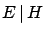can have three values:

TRUE:
ifis TRUE andis TRUE;
FALSE:
ifis FALSE andis TRUE;
UNDETERMINED:
ifis FALSE; in this case we are merely uninterested in what happens to. In terms of betting, the bet is invalidated and none loses or gains.
Then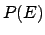can be written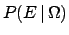, to state explicitly that it is the probability ofwhatever happens to the rest of the world (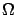means all possible events). We realize immediately that this condition is really too vague and nobody would bet a penny on a such a statement. The reason for usually writingis that many conditions are implicitly, and reasonably, assumed in most circumstances. In the classical problems of coins and dice, for example, one assumes that they are regular. In the example of the energy loss, it was implicit (obvious'') that the high voltage was on (at which voltage?) and that HERA was running (under which condition?). But one has to take care: many riddles are based on the fact that one tries to find a solution which is valid under more strict conditions than those explicitly stated in the question, and many people make bad business deals by signing contracts in which what was obvious'' was not explicitly stated.

In order to derive the formula of conditional probability let us assume for a moment that it is reasonable to talk about absolute probability''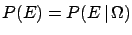, and let us rewrite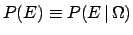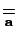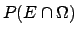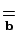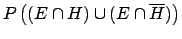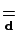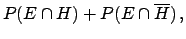(3.1)

where the result has been achieved through the following steps:
(a)implies(i.e.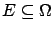) and hence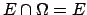;
(b)
the complementary eventsand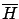make a finite partition of, i.e.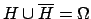;
(c)
distributive property;
(d)
axiom 3.
The final result of () is very simple:is equal to the probability thatoccurs andalso occurs, plus the probability thatoccurs butdoes not occur. To obtainwe just get rid of the subset ofwhich does not contain(i.e.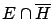) and renormalize the probability dividing by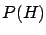, assumed to be different from zero. This guarantees that if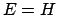then. We get, finally, the well known formula(3.2)

In the most general (and realistic) case, where bothandare conditioned by the occurrence of a third event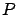, the formula becomes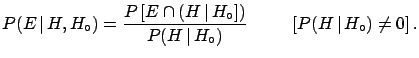(3.3)

Usually we shall make use of () (which means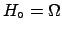) assuming thathas been properly chosen. We should also remember that () can be resolved with respect to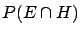, obtaining the well known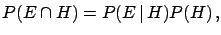(3.4)

and by symmetry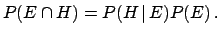(3.5)

We remind that two events are called independent if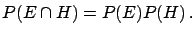(3.6)

This is equivalent to saying that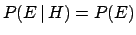and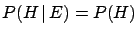, i.e. the knowledge that one event has occurred does not change the probability of the other. If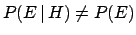then the eventsandare correlated. In particular:
• if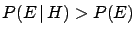thenandare positively correlated;
• if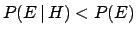thenandare negatively correlated.Next: Bayes' theorem Up: Conditional probability and Bayes' Previous: Dependence of the probability   Contents
Giulio D'Agostini 2003-05-15